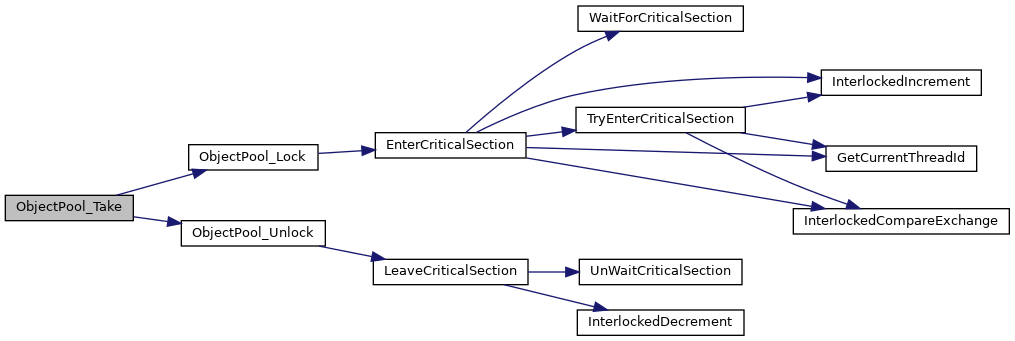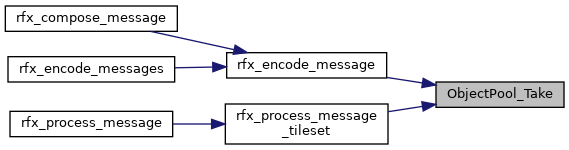FreeRDP
ObjectPool.c File Reference
`#include <winpr/crt.h>`
`#include <winpr/collections.h>`

Functions

voidObjectPool_Take (wObjectPool *pool)

void ObjectPool_Return (wObjectPool *pool, void *obj)

void ObjectPool_Clear (wObjectPool *pool)

wObjectPool * ObjectPool_New (BOOL synchronized)

void ObjectPool_Free (wObjectPool *pool)

Function Documentation

 void ObjectPool_Clear ( wObjectPool * pool )

Releases the buffers currently cached in the pool.

Here is the call graph for this function: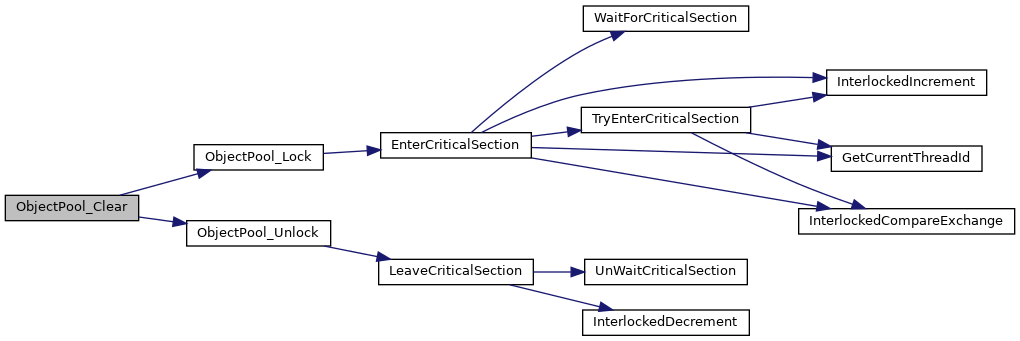Here is the caller graph for this function: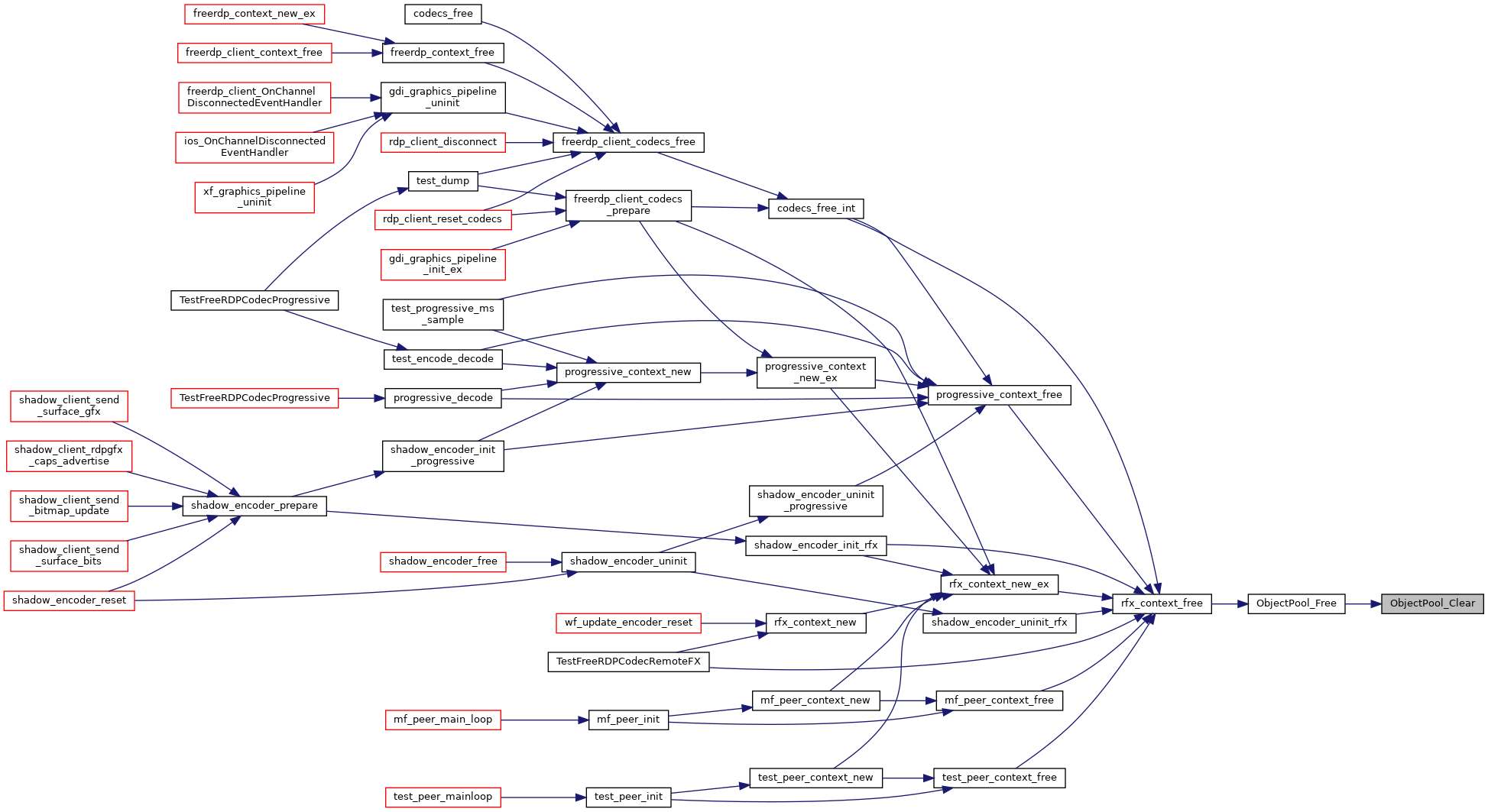void ObjectPool_Free ( wObjectPool * pool )

Here is the call graph for this function: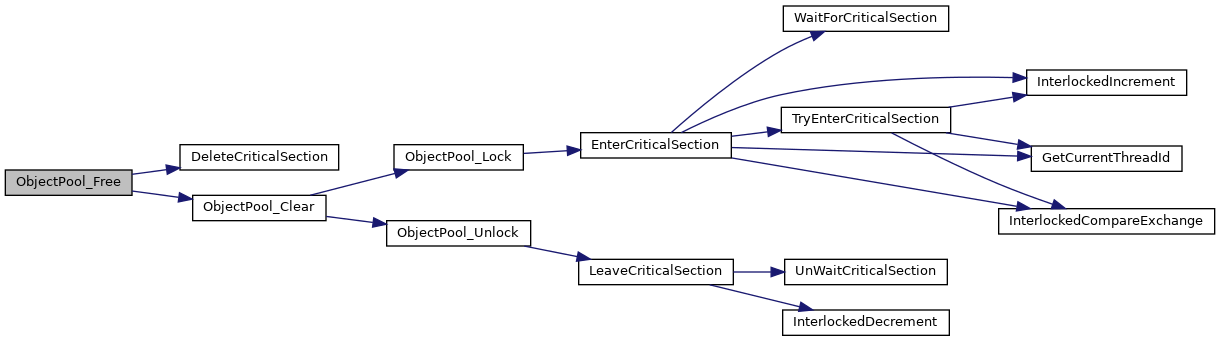Here is the caller graph for this function: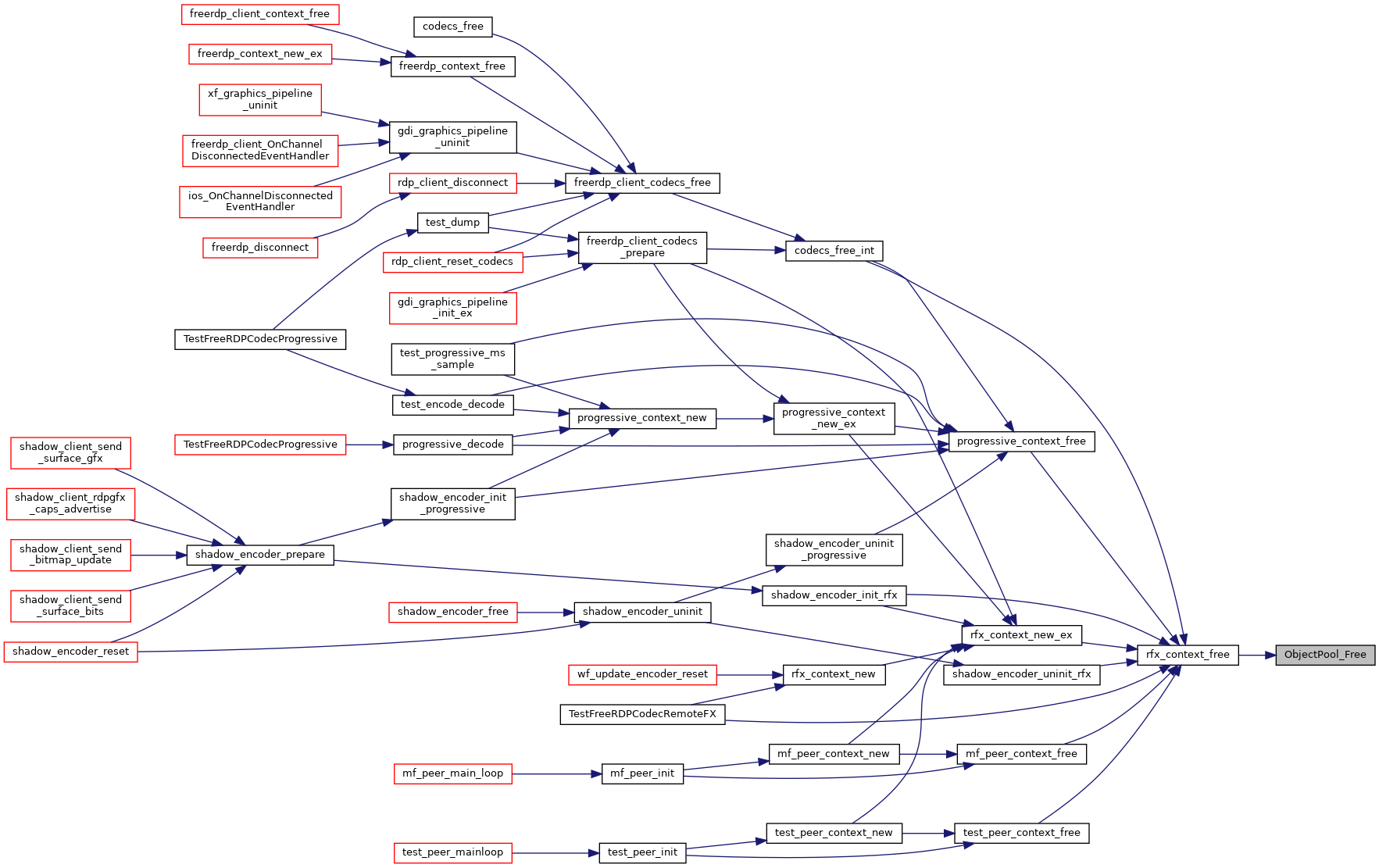wObjectPool* ObjectPool_New ( BOOL synchronized )

Construction, Destruction

Here is the call graph for this function: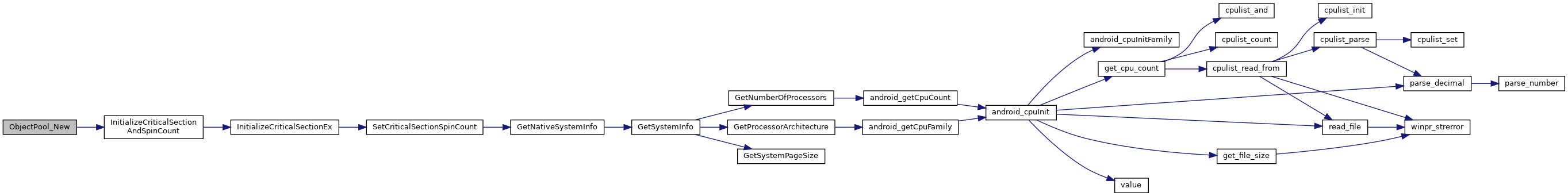Here is the caller graph for this function: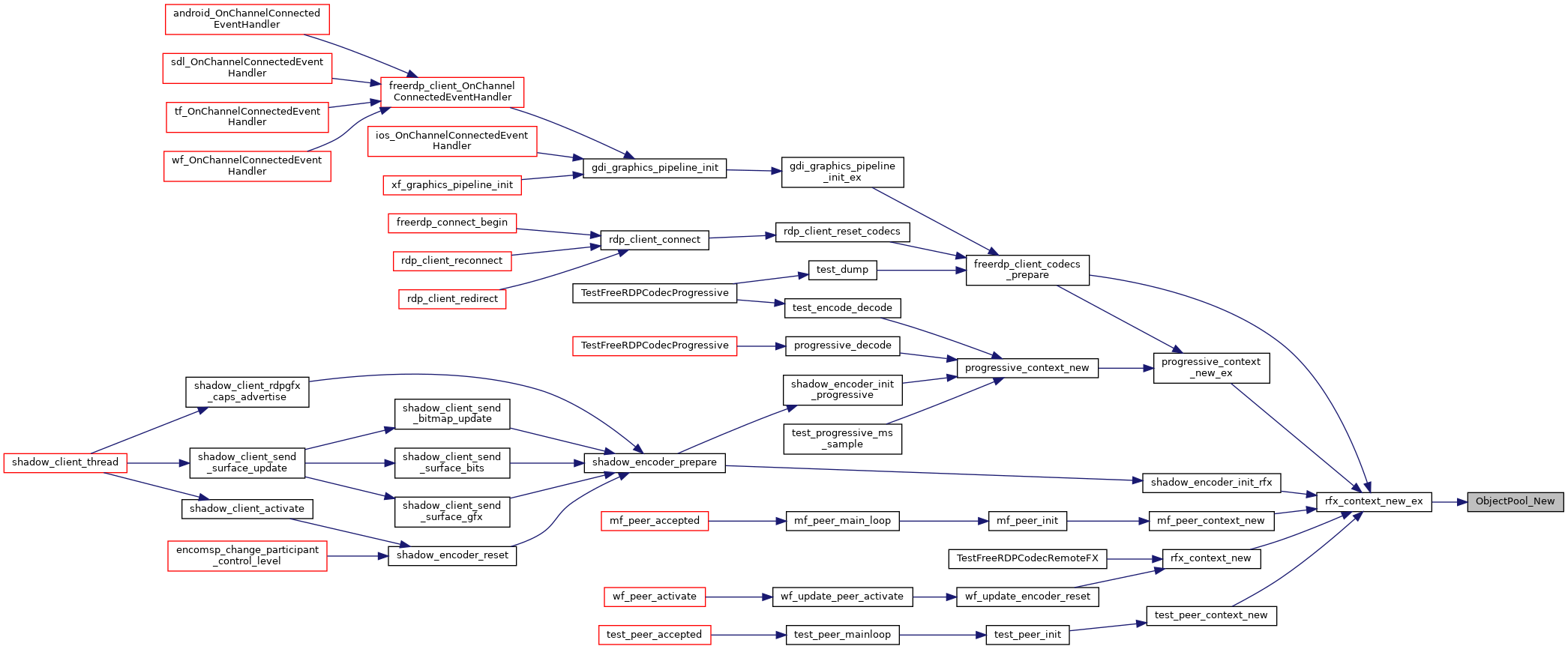void ObjectPool_Return ( wObjectPool * pool, void * obj )

Returns an object to the pool.

Here is the call graph for this function: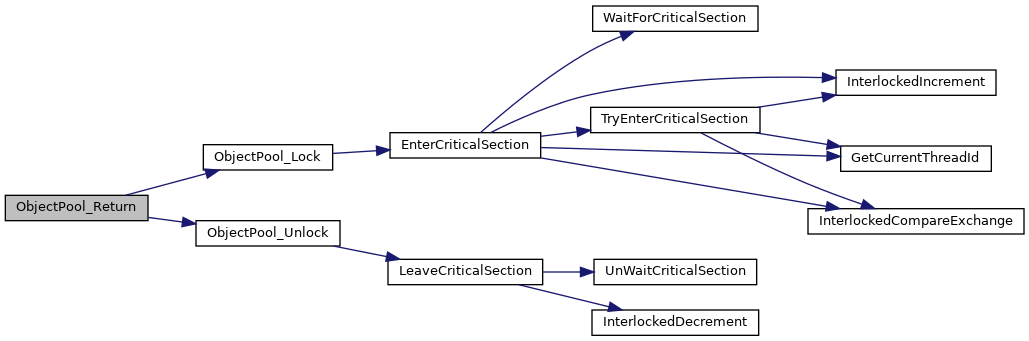Here is the caller graph for this function: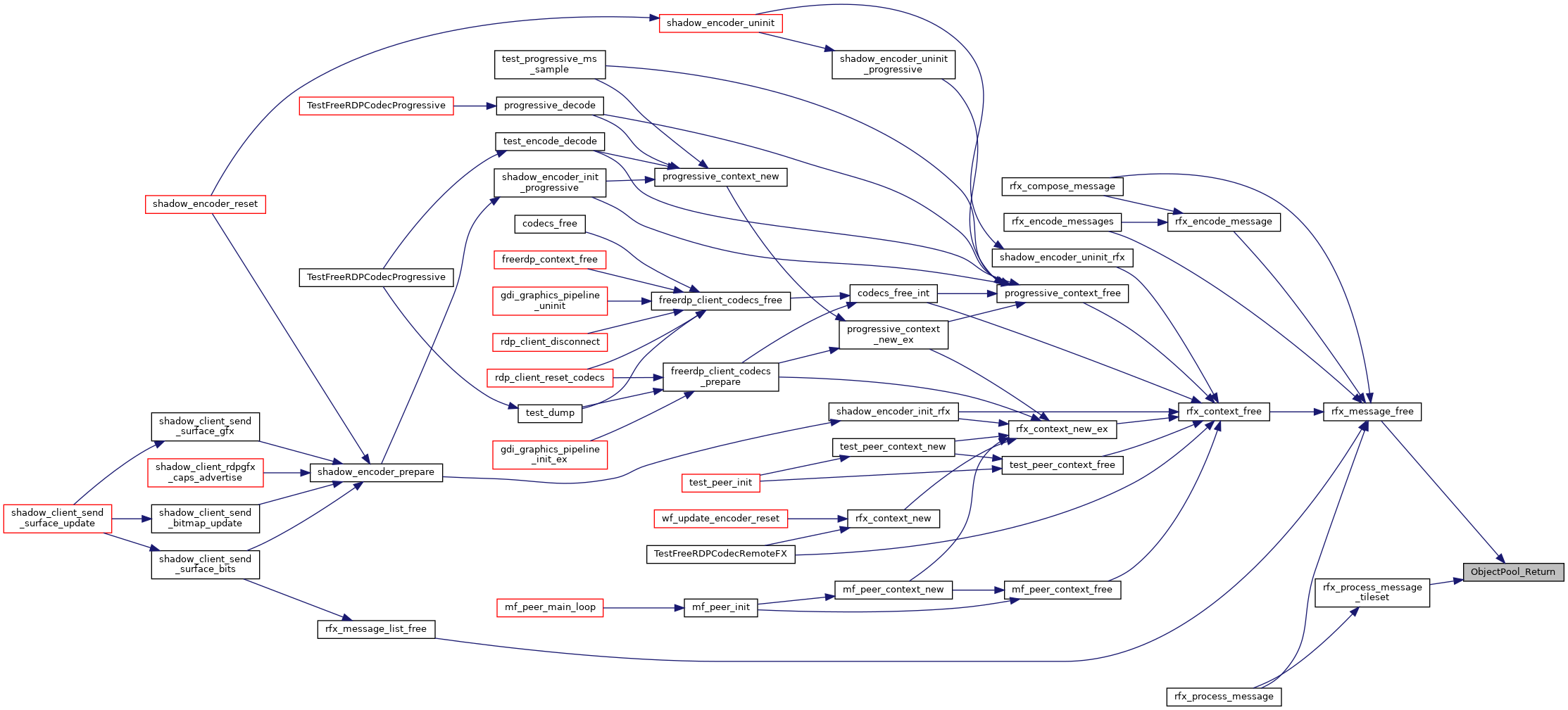void* ObjectPool_Take ( wObjectPool * pool )

WinPR: Windows Portable Runtime Object Pool

```http://www.apache.org/licenses/LICENSE-2.0